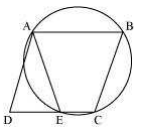# ABCD is a parallelogram. The circle through A, B and C intersect CD (produced if necessary) at E. Prove that AE, = AD.#### Solution

Given

ABCD is a ||gm. The  circle through A,B and C intersect at E.

To prove:

Proof:

∠ABC+∠AEC=180°      —–[sum of opposite is of a cyclic quadrilateral is 180°]

∠AEC=180°-∠EAB  ————(i)

Now ∠AEC+∠DAE=180°-∠DAE ————(ii)

From 1 and 2

180°-∠ABC=∠180°-∠DAE

∠ABC=∠DAE   ————(iii)

Also ∠ABC=∠DAE       ————(iv)

From 3 and 4

∴AE=AD        ——[sides apposite to equal angles in  ∆ are equal]

Hence Proved